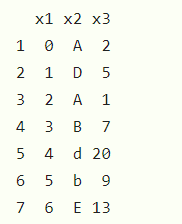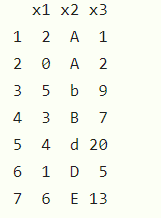# Sort a given DataFrame by multiple column(s) in R

Sorting of data may be useful when working on a large data and data is un-arranged, so it is very helpful to sort data first before applying operations. In this article, we will learn how to sort given dataframes by multiple columns in R.

### Approach:

• Create data frame
• Choose any more number of columns more than one
• Pass those columns as the parameter in the sorting functions.
• Display result

Data frame in use:### Method 1: Using order() and with()

with() is used to evaluate an expression that is related to some data

Syntax:

with(data, expression)

order() is used to order the vectors given to it

Syntax:

order(vector(s))

Example:

## R

 `data <- ``data.frame``(x1 = 0:6,                          ` `                 ``x2 = ``c``(``"A"``, ``"D"``, ``"A"``, ``"B"``, ``"d"` `, ``"b"` `, ``"E"``), ` `                 ``x3 = ``c``(2, 5, 1, 7, 20, 9 , 13)) ` `data ` ` `  `data[``with``(data, ``order``(x2, x3)), ]`

Output:### Method 2: Using arrange()

Syntax: Arrange()

Parameter:

• dataframe: The dataframe on which we want to sort.
• x1, x2:  These at the sorting columns.

This function is placed in the “dplyr” package so first, we have to install it explicitly.

## R

 `# Load dplyr package ` `library``(``"dplyr"``) ` ` `  `# Create example data ` `data <- ``data.frame``(x1 = 0:6, ` `                 ``x2 = ``c``(``"A"``, ``"D"``, ``"A"``, ``"B"``, ``"d"` `, ``"b"` `, ``"E"``), ` `                 ``x3 = ``c``(2, 5, 1, 7, 20, 9 , 13)) ` `data ` `arrange``(data, x2, x3)`

Output:Whether you're preparing for your first job interview or aiming to upskill in this ever-evolving tech landscape, GeeksforGeeks Courses are your key to success. We provide top-quality content at affordable prices, all geared towards accelerating your growth in a time-bound manner. Join the millions we've already empowered, and we're here to do the same for you. Don't miss out - check it out now!